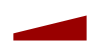Reflexxes Motion Libraries  Manual and Documentation (Type II, Version 1.2.6)
Description of Output Values

After calling the position-based On-Line Trajectory Generation (OTG) algorithm with ReflexxesAPI::RMLPosition(), the output values can be read from the class RMLPositionOutputParameters. The output values of the velocity-based On-Line Trajectory Generation algorithm, which is called with ReflexxesAPI::RMLVelocity() can be read from the class RMLVelocityOutputParameters. For a detailed description of these classes, please refer to the class documentation, and for a description of the input values of the Reflexxes Motion Library, please refer to the Description of Input Values.

This page contains two main sections with one example each:

## Output Values of the Position-based On-Line Trajectory Generation AlgorithmInput and output values of the Reflexxes Type II Motion Library in a generic manner.

The position-based Type II On-Line Trajectory Generation algorithm called at a time instant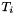computes the following output values (cf. RMLPositionOutputParameters):

See also:
RMLPositionOutputParameters
Description of Input Values

## Example

The position-based On-Line Trajectory Generation algorithm of the Type II Reflexxes Motion Library is called by the method ReflexxesAPI::RMLPosition().

In this example (cf. Example 1 — Introduction to the Position-based algorithm), we assume to control a simple Cartesian robot with three translational degrees of freedom, and we apply the following input values at time instant.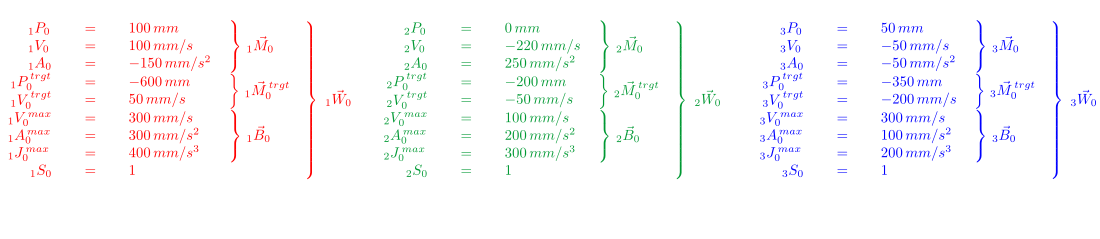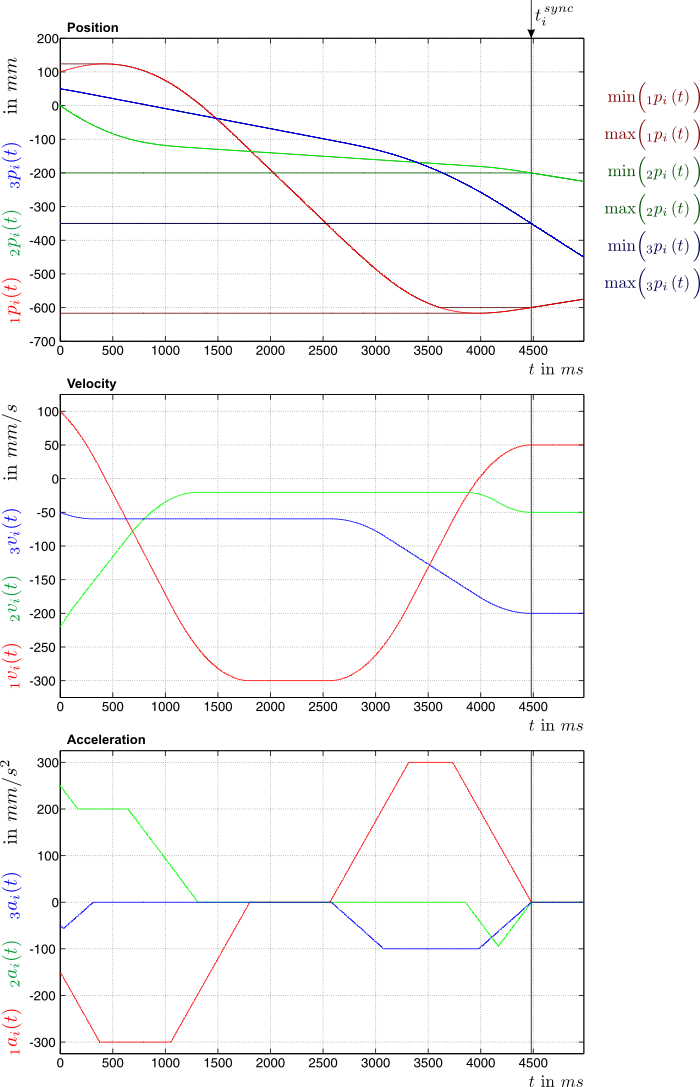Sample trajectory generated by the position-based Type II On-Line Trajectory Generation algorithm of the Reflexxes Motion Libraries.

Further examples can be found on pages

Note:
The input variables

are only used by the Type IV Reflexxes Motion Library.

## Sample Source Code

For a program to set-up the corresponding input parameters please refer to the Example 1 — Introduction to the Position-based algorithm, to the Example 2 — Making Use of Output Parameters of the Position-based algorithm, and to the Screen Output of 02_RMLPositionSampleApplication.cpp.

## Output Values of the Velocity-based On-Line Trajectory Generation Algorithm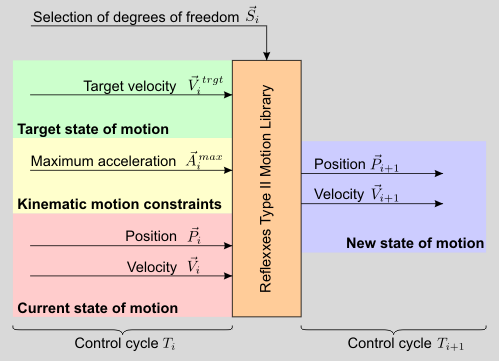Input and output values of the velocity-based Type II On-Line Trajectory Generation algorithm of the Reflexxes Motion Libraries.

The velocity-based Type II On-Line Trajectory Generation algorithm is executed by a call of ReflexxesAPI::RMLVelocity(), and its output values RMLVelocityOutputParameters are the very same as for the position-based algorithm (see above), but the values for the target position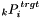and the maximum velocity vector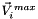are not considered, and the flag RMLPositionFlags::KeepCurrentVelocityInCaseOfFallbackStrategy is not available.

See also:
RMLVelocityOutputParameters
Description of Input Values

Note:
The velocity-based algorithm is also used in the second layer of the safety mechanism to guarantee valid output value for any given set of input values (cf. Safety: Three Layers of Error Handling).

## Example

For this second example (cf. Example 4 — Introduction to the Velocity-based algorithm), we again assume a very simply Cartesian robot with three translational degrees of freedom.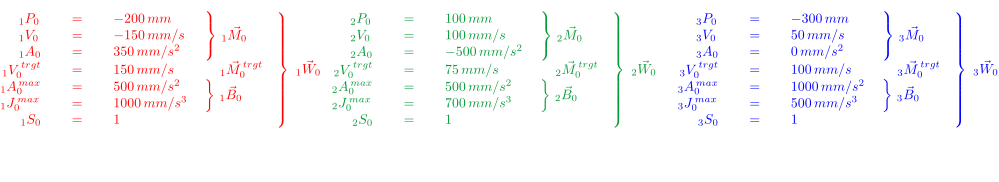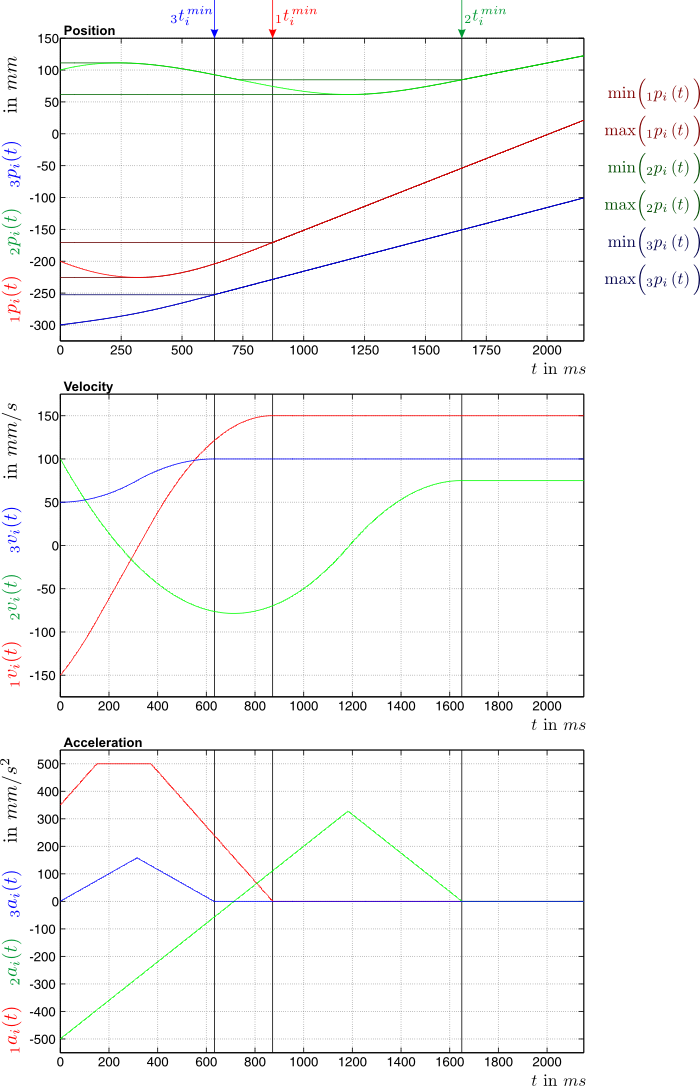Sample trajectory generated by the velocity-based Type II On-Line Trajectory Generation algorithm of the Reflexxes Motion Libraries.

## Sample Source Code

For a program to set-up the corresponding input parameters please refer to the Example 4 — Introduction to the Velocity-based algorithm, to the Example 5 — Making Use of Output Parameters of the Velocity-based algorithm, and to the Screen Output of 05_RMLVelocitySampleApplication.cpp.

User documentation of the Reflexxes Motion Libraries by Reflexxes GmbH (Company Information, Impressum). This document was generated with Doxygen on Mon Jul 7 2014 13:21:09. Copyright 2010–2014.